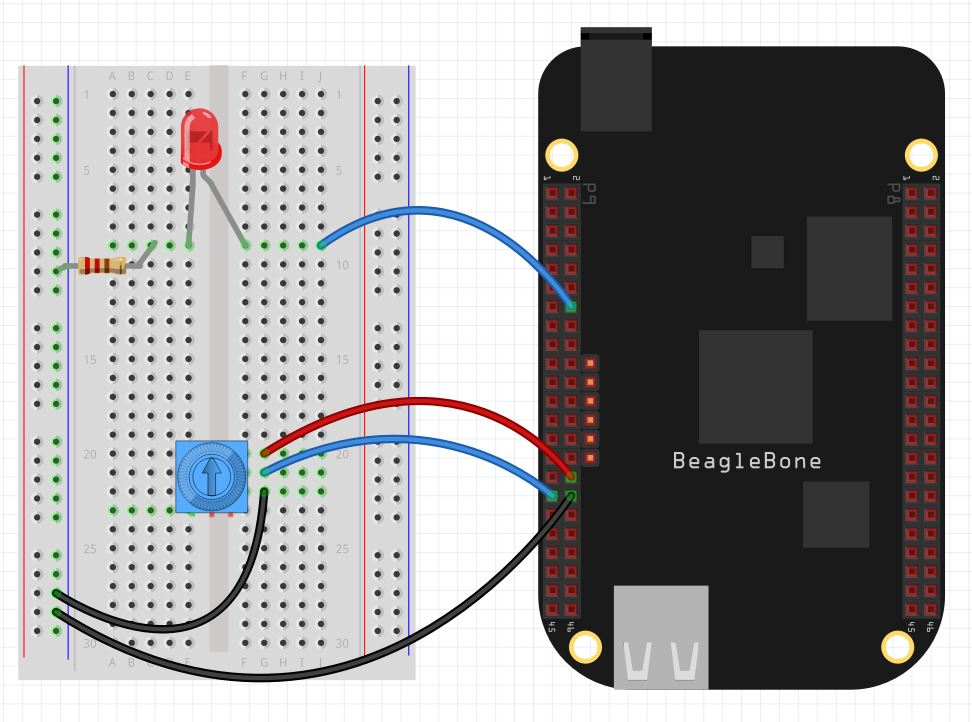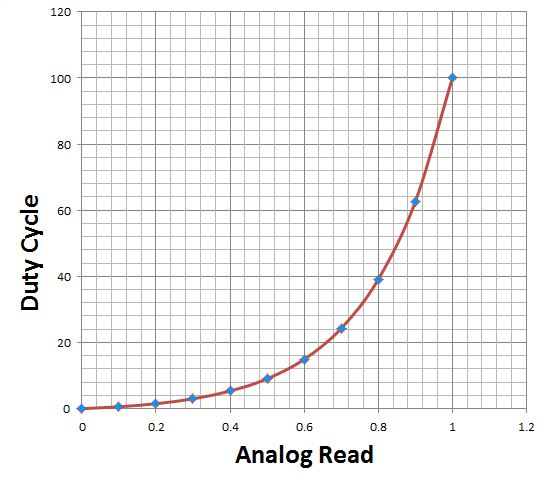# Beaglebone Black LESSON 10: Dimable LED using Potentiometer

In this lesson we will create a dimable LED. We will read an analog voltage from a potentiometer, and use that to set the brightness on an LED. In order to proceed with this lesson, you will need to connect the following circuit:Potentiometer Reading is Used to Set LED Brightness

Note we are using P9_32 as the reference voltage on the voltage divider, we are using P9_34 as the reference ground, and we are using P9_33 as the analog sense pin. We also using P9_14 as the PWM output pin. Note the current limiting resistor in series with the LED is 330 Ohm.

The object of this circuit is to read the value of the potentiometer and then to use that to set the brightness on the LED. We know that the value we read from the potentiometer will be between 0 and 1. We know that what we can control on the PWM pin is the duty cycle of the 3.3 volt signal. We know that when the potentiometer reads 0, we want a 0% duty cycle on the PWM pin, which would have the LED off. This is our first point:

(0,0)

We also know that when we read 1 from the potentiometer, we want to apply a duty cycle of 100%, or have the LED be full bright. This is our second point:

(1,100)

If we created an equation for the line between these two points, we could calculate the duty cycle that should be applied based on the potentiometer reading. The problem with this is the way our eye perceives changes in brightness. We perceive exponential changes, so if we connected the two points with a linear relationship we would see lots of change at the low end of the scale, but as we continued to move the potentiometer, the brightness would appear to saturate. In order to have a nice smooth transition from full dim to full bright as the potentiometer is moved from left to right, we need to fit an exponential curve between the two points above. We want the LED to be off when the pot is full left, and full bright when the pot is fully to the right. We could use the following exponential equation:

Duty Cycle = C^(Analog Read) – B

This should do the trick, but we need to figure out what the constants C and B need to be. We do this by first plugging in the first point (0,0) from above:

0 = C^0 – B

Anything raised to 0 power is 1, so we have:

0= 1 – B

So B = 1. There, we have our first constant. We use this, and our second point to find C.

100 = C^1 – 1

101=C^1

C=101

Now we have everything we need to calculate the Duty Cycle from the value we read from the potentiometer. The final equation is:

Duty Cycle = 101^(Analog Read) – 1This Chart Shows how to Map Duty Cycle onto Analog Read

Note that this relationship has the desired properties. When we read a 0 from the potentiometer, we apply a Duty Cycle of 0% to the PWM pin, and the LED is off. When we read a 1 from the potentiometer, we apply a Duty Cycle of 100% to the LED and it is full bright. The exponential shape of the curve between these two points ensures that we will perceive a smooth increase in brightness as we turn the potentiometer up. Math works! It would be very hard to do this by trial and error.

We are now ready to begin developing our code. The video lesson explains the code line-by-line, and we are using commands we learned in the last few lessons.

The code works very well, and produces a very smooth transition from fully off to fully bright.